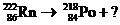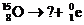Chapter 19.1, Problem 19.2SC### Introductory Chemistry: A Foundati...

9th Edition
Steven S. Zumdahl + 1 other
ISBN: 9781337399425

#### Solutions

Chapter
Section### Introductory Chemistry: A Foundati...

9th Edition
Steven S. Zumdahl + 1 other
ISBN: 9781337399425
Textbook Problem
1 views

# Exercise 19.2 Supply the missing species in each of the following nuclear equations.ab.Interpretation Introduction

(a)

Interpretation:

Interpret and complete the following reaction.

86Rn222   84Po218 + x.

Concept Introduction:

The decaying of an atom takes place by three type of decay such as alpha decay, beta decay, gama decay.

In alpha decay, an alpha particle with mass 4m and electron 2e removed from nuclide while in beta decay two type of decay are possible beta (+) and beta (-). In gamma decay, nuclide will remain same to the original one.

Explanation

Alpha particle decaying of Rn results in formation of polonium with mass 218 as follows:

86Rn222

Interpretation Introduction

(b)

Interpretation:

Interpret and complete the following reaction.

8O15   x +1e0.

Concept Introduction:

The decaying of an atom takes place by three type of decay such as alpha decay, beta decay, gama decay.

In alpha decay, an alpha particle with mass 4m and electron 2e removed from nuclide while in beta decay two type of decay are possible beta (+) and beta (-). In gamma decay, nuclide will remain same to the original one.

### Still sussing out bartleby?

Check out a sample textbook solution.

See a sample solution

#### The Solution to Your Study Problems

Bartleby provides explanations to thousands of textbook problems written by our experts, many with advanced degrees!

Get Started